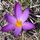cancel
Showing results for
Did you mean:Resolver I

## Looping throughout a list of tables

Hi,

I have a list of tables with an unknown number of items: "SpreadsheetList"

I have to consolidate all the tables in a sigle table e.g. with

but, before to combine them, I have to perform two transformations on each of them, which are:

#"Unpivoted Other Columns" = Table.UnpivotOtherColumns(#"Promoted Headers", {"Col1", "Col2", "Col3"}, "Attribute", "Value")

unfortunately I don't know how to perform these 2 transformations on each item of the list before to combine the result in a single table.

Thank you for any help.
m
1 ACCEPTED SOLUTIONSuper User

You could create a function out of the applied steps but, in this case, it might be simpler to just smash everything together in one step like this:

``````BigTable =
Table.Combine(
List.Transform(
Table.UnpivotOtherColumns(
{"Col1", "Col2", "Col3"},
"Attribute", "Value"
)
)
)``````

2 REPLIES 2Super User

You could create a function out of the applied steps but, in this case, it might be simpler to just smash everything together in one step like this:

``````BigTable =
Table.Combine(
List.Transform(
Table.UnpivotOtherColumns(
{"Col1", "Col2", "Col3"},
"Attribute", "Value"
)
)
)``````Resolver I

Thank you so much @AlexisOlson, your SOLUTION is just perfect!!!

At the end, I implemented it with a function (so you can check if it's OK ;-))

Here is the full code which exctracts some specific Excel files from a Sharepoint url,

perform some transformations on each spreadsheet of each workbook,

and put the result in a single table.

``````let
Source = SharePoint.Files("my Sharepoint url", [ApiVersion = 15]),
#"Filtered Rows" = Table.SelectRows(Source, each Text.StartsWith([Name], "my text filter")),

WorkbookList = List.Transform(#"Filtered Rows"[Content], each Excel.Workbook(_)[Data]),

TransformTable = (SpreadsheetList as list) as list =>
let
TransformedTable = List.Transform(
Table.UnpivotOtherColumns(
{"Col1", "Col2", "Col3"},
"Attribute", "Value"))
in
TransformedTable,

in

Thank you again for your solution

m